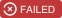vt-pbf

Serialize mapbox vector tiles to binary protobufs in javascript.

Usage no npm install needed!

<script type="module">
import vtPbf from 'https://cdn.skypack.dev/vt-pbf';
</script>

vt-pbfSerialize Mapbox vector tiles to binary protobufs in javascript.

Usage

As far as I know, the two places you might get a JS representation of a vector tile are geojson-vt and vector-tile-js. These both use slightly different internal representations, so serializing each looks slightly different:

From vector-tile-js

var vtpbf = require('vt-pbf')
var VectorTile = require('@mapbox/vector-tile').VectorTile
var Protobuf = require('pbf')

var data = fs.readFileSync(__dirname + '/fixtures/rectangle-1.0.0.pbf')
var tile = new VectorTile(new Protobuf(data))
var orig = tile.layers['geojsonLayer'].feature(0).toGeoJSON(0, 0, 1)

var buff = vtpbf(tile)
fs.writeFileSync('my-tile.pbf', buff)

From geojson-vt

var vtpbf = require('vt-pbf')
var geojsonVt = require('geojson-vt')

var orig = JSON.parse(fs.readFileSync(__dirname + '/fixtures/rectangle.geojson'))
var tileindex = geojsonVt(orig)
var tile = tileindex.getTile(1, 0, 0)

// pass in an object mapping layername -> tile object
var buff = vtpbf.fromGeojsonVt({ 'geojsonLayer': tile })
fs.writeFileSync('my-tile.pbf', buff)

vtpbf.fromGeojsonVt takes two arguments:

• layerMap is an object where keys are layer names and values are a geojson-vt tile,
• options is an object (optional argument). There are 2 supported keys: version to define the version of the mvt spec used and extent to define the extent of the tile. version defaults to 1 and extent to 4096.